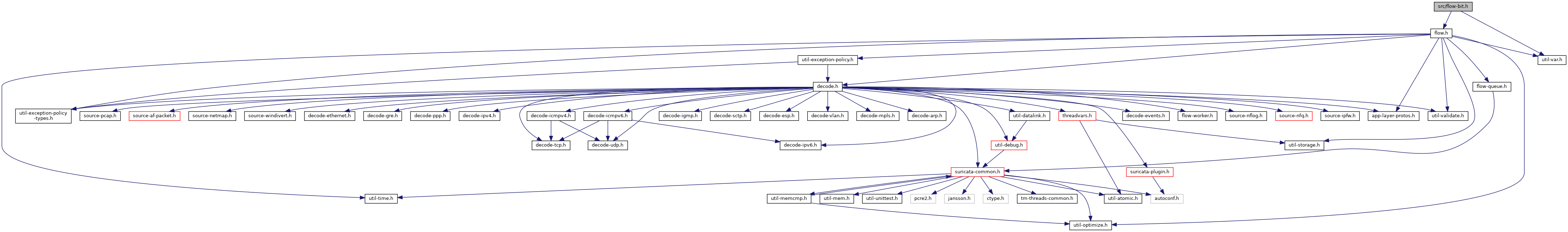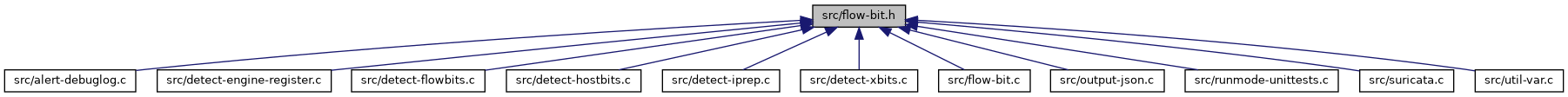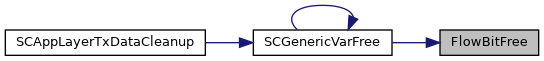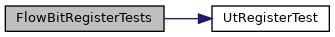suricata
flow-bit.h File Reference
`#include "flow.h"`
`#include "util-var.h"`
Include dependency graph for flow-bit.h:This graph shows which files directly or indirectly include this file:Go to the source code of this file.

struct  FlowBit_

## Typedefs

typedef struct FlowBit_ FlowBit

## Functions

void FlowBitFree (FlowBit *)

void FlowBitRegisterTests (void)

void FlowBitSet (Flow *, uint32_t)

void FlowBitUnset (Flow *, uint32_t)

void FlowBitToggle (Flow *, uint32_t)

int FlowBitIsset (Flow *, uint32_t)

int FlowBitIsnotset (Flow *, uint32_t)

## Detailed Description

Definition in file flow-bit.h.

## Typedef Documentation

 typedef struct FlowBit_ FlowBit

## Function Documentation

 void FlowBitFree ( FlowBit * )

Definition at line 128 of file flow-bit.c.

References Flow_::flowvar, GenericVarFree(), and SCFree.

Referenced by GenericVarFree().

Here is the call graph for this function:Here is the caller graph for this function:int FlowBitIsnotset ( Flow * , uint32_t )

Definition at line 116 of file flow-bit.c.

Referenced by DetectFlowbitsRegister().

Here is the caller graph for this function:int FlowBitIsset ( Flow * , uint32_t )

Definition at line 104 of file flow-bit.c.

Referenced by DetectFlowbitsRegister().

Here is the caller graph for this function:void FlowBitRegisterTests ( void )

Definition at line 402 of file flow-bit.c.

References UtRegisterTest().

Here is the call graph for this function:void FlowBitSet ( Flow * , uint32_t )

Definition at line 84 of file flow-bit.c.

Referenced by DetectFlowbitsRegister().

Here is the caller graph for this function:void FlowBitToggle ( Flow * , uint32_t )

Definition at line 94 of file flow-bit.c.

Referenced by DetectFlowbitsRegister().

Here is the caller graph for this function:void FlowBitUnset ( Flow * , uint32_t )

Definition at line 89 of file flow-bit.c.

Referenced by DetectFlowbitsRegister().

Here is the caller graph for this function: